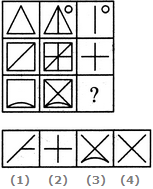# Non Verbal Reasoning - Figure Matrix - Discussion

### Discussion :: Figure Matrix - Section 1 (Q.No.1)

In each of the following questions, find out which of the answer figures (1), (2), (3) and (4) completes the figure matrix ?

1.

Select a suitable figure from the four alternatives that would complete the figure matrix.[A]. 1 [B]. 2 [C]. 3 [D]. 4

Explanation:

The third figure in each row comprises of parts which are not common to the first two figures.

 Jayant said: (Sep 21, 2012) How I solve it in few minutes.

 Kifayat Ullah Shah said: (Jun 19, 2013) The Middle figure in each row is combination of left and right figure.

 Polash said: (Mar 21, 2014) How can I understand this is the answer. Is their any clue or descriptive solution?

 Jangya said: (Jul 10, 2014) What is the meaning of problem figures, how can I solved?

 Keshaw said: (Oct 22, 2014) To get the figures in the third column of the matrix look for the part of the figures which is not common in column one and two of the matrix.

 Kanu Priya said: (Nov 26, 2014) How to solve these problems quickly?

 Shanti said: (Mar 24, 2015) Picture is remove in every step.

 Dine said: (May 26, 2015) This is so confusing why is it 4 I am going to pick 4.

 Shashank Shrey said: (Oct 17, 2016) Those who have a doubt to this question, Please listen- There are 3 boxes given. 1) Observe the first figure and the second figure. 2) In the second figure, there is an extra figure added to the first figure. 3) The figure added in the first figure to make the second figure is given in the third place.

 Jamie said: (Dec 5, 2016) Thank you all.

 Priyanka said: (Dec 9, 2016) Thank you all, for the explanation.

 Sonia Gupta said: (Dec 11, 2016) Thank you all for giving the explanation.

 Rakib said: (Apr 15, 2017) Thanks lot @Shashank Shrey.

 Adarsh Ns said: (Feb 7, 2019) Thanks you for giving the explanation @Shashank shrey.

 Sandhya said: (Feb 24, 2021) Thank you @Shashank.

 Makhan Lal said: (Jul 4, 2021) How to solve this? Please anyone explain.